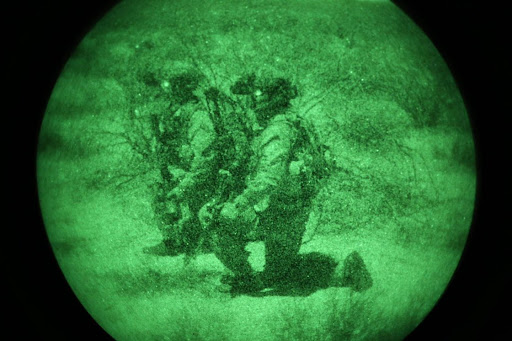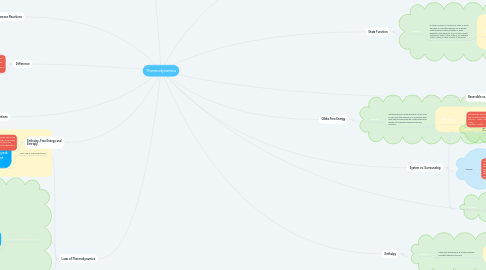# ThermodynamicsGabriel Hipolito

Chemistry "Thermodynamics" submission

Get Started. It's FreeThermodynamics## 2. Laws of Thermodynamics

### 2.1. First Law of Thermodynamics

2.1.1. The first law of thermodynamics, also known as Law of Conservation of Energy, states that energy can neither be created nor destroyed; energy can only be transferred or changed from one form to another.

2.1.1.1. This law says that there are two kinds of processes, heat and work, that can lead to a change in the internal energy of a system. Since both heat and work can be measured and quantified, this is the same as saying that any change in the energy of a system must result in a corresponding change in the energy of the surroundings outside the system.

2.1.1.1.1. In other words, energy cannot be created or destroyed. If heat flows into a system or the surroundings do work on it, the internal energy increases and the sign of q and w are positive. Conversely, heat flow out of the system or work done by the system (on the surroundings) will be at the expense of the internal energy, and q and w will therefore be negative.

2.1.1.2. ΔU=q+w

### 2.2. Second Law of Thermodynamics

2.2.1. The second law of thermodynamics says that the entropy of any isolated system always increases.

2.2.1.1. Isolated systems spontaneously evolve towards thermal equilibrium—the state of maximum entropy of the system. More simply put: the entropy of the universe (the ultimate isolated system) only increases and never decreases.

2.2.1.1.1. Example: a room, if not cleaned and tidied, will invariably become more messy and disorderly with time – regardless of how careful one is to keep it clean. When the room is cleaned, its entropy decreases, but the effort to clean it has resulted in an increase in entropy outside the room that exceeds the entropy lost.

2.2.1.2. ΔSuniv=ΔSsys+ΔSsurr≥0

2.2.1.3. Second Law of Thermodynamics

### 2.3. Third Law of Thermodynamics

2.3.1. The third law of thermodynamics states that the entropy of a system approaches a constant value as the temperature approaches absolute zero.

2.3.1.1. The entropy of a system at absolute zero is typically zero, and in all cases is determined only by the number of different ground states it has

2.3.1.1.1. Specifically, the entropy of a pure crystalline substance (perfect order) at absolute zero temperature is zero. This statement holds true if the perfect crystal has only one state with minimum energy.

2.3.1.2. Third Law of Thermodynamics

## 5. Enthalpy, Free Energy and Entropy

### 5.1. Gibbs free energy is the energy associated with a chemical reaction that can do useful work. It equals the enthalpy minus the product of the temperature and entropy of the system.

5.1.1. At constant temperature ΔG = ΔH – TΔS

5.1.1.1. ΔG predicts the direction of a chemical reaction. If ΔG is negative, then the reaction is spontaneous. If ΔG is positive, then the reaction is non-spontaneous.

## 7. Gibbs Free Energy

### 7.1. Definition

7.1.1. a thermodynamic potential that can be used to calculate the maximum of reversible work that may be performed by a thermodynamic system at a constant temperature and pressure.

7.1.1.1. Analogy

7.1.1.1.1. Estimate the temperature where ΔG = 0 for the following reaction: (Given: ΔH = -176 kJ and ΔS = -284.5 J/K) CAUTION: Beware of units. NH3(g) + HCl(g) ---> NH4Cl(s)

## 8. Enthalpy

### 8.1. Definition

8.1.1. is the heat absorbed by a system during a constant-pressure process.

8.1.1.1. Analogy

## 9. Entropy

### 9.1. Definition

9.1.1. a measure of the randomness (disorder) in a system (state function)

9.1.1.1. Analogy

9.1.1.1.1. Take a campfire for example. The solid wood burns and becomes ash, smoke and gases, all of which spread energy outwards more easily than the solid fuel

## 10. System vs. Surrounding

### 10.1. System

10.1.1. The collection of matter that is being studied

### 10.2. Analogy

10.2.1. Take a thermos for example. The inner chamber of the thermos, where liquid is stored, is the system. The surrounding, then, would be the outer layers of the thermos, that work to keep energy in the thermos, but always absorb some of the thermos' energy eventually.

### 10.3. Surrounding

10.3.1. Anything that is surrounding the system

## 11. Reversible vs. Irreversible

### 11.1. Reversible

11.1.1. Reversible processes involve no change in entropy, so they can be reversed with no extra energy input without breaking the Second Law of Thermodynamics

11.1.1.1. Analogy

11.1.1.1.1. Heat transfer between a reservoir and a system is an externally reversible process if the surface of contact between the system and reservoir is at the same temperature

### 11.2. Irreversible

11.2.1. Irreversible processes increase entropy of their surroundings. If you were to put everything back the way it was, there must still have been an increase in entropy somewhere in the universe that makes ΔS ≥ 0.

11.2.1.1. Analogy

11.2.1.1.1. An egg falling on the floor and cracking open, spilling its contents in the process.

## 12. State Function

### 12.1. Definition

12.1.1. A state function or function of state or point function is a function defined for a system relating several state variables or state quantities that depends only on the current equilibrium state of the system, for example a gas, a liquid, a solid, crystal, or emulsion.

12.1.1.1. Analogy

12.1.1.1.1. Imagine you are attempting to scale a 4,000 foot mountain. You can either hike, bike, or ride a train to reach the peak. Despite all; three of these options involving varying amounts of energy, the end result will always be the same - you will reach the peak of the mountain. This is an example of a state function, because no matter how you got to the top, you still scaled 4,000 feet.

## 13. Work

### 13.1. Definition

13.1.1. Work is defined as the quantity of energy that is transferred from one system to another.

13.1.1.1. Analogy

13.1.1.1.1. When an individual strikes a punching bag, they are transferring energy from their fist and onto the punching bag. This transfer of energy is known as "work."